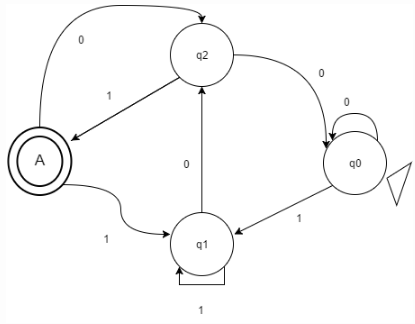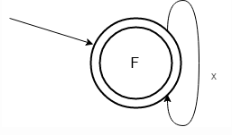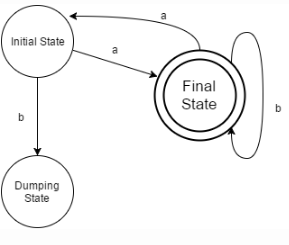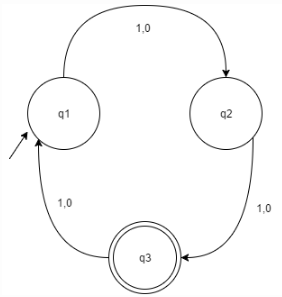Courses

# Test: Deterministic Finite Automata: Introduction & Definition

## 10 Questions MCQ Test Theory of Computation | Test: Deterministic Finite Automata: Introduction & Definition

Description
This mock test of Test: Deterministic Finite Automata: Introduction & Definition for Computer Science Engineering (CSE) helps you for every Computer Science Engineering (CSE) entrance exam. This contains 10 Multiple Choice Questions for Computer Science Engineering (CSE) Test: Deterministic Finite Automata: Introduction & Definition (mcq) to study with solutions a complete question bank. The solved questions answers in this Test: Deterministic Finite Automata: Introduction & Definition quiz give you a good mix of easy questions and tough questions. Computer Science Engineering (CSE) students definitely take this Test: Deterministic Finite Automata: Introduction & Definition exercise for a better result in the exam. You can find other Test: Deterministic Finite Automata: Introduction & Definition extra questions, long questions & short questions for Computer Science Engineering (CSE) on EduRev as well by searching above.
QUESTION: 1

### Which of the following not an example Bounded Information?

Solution:

Bounded information refers to one whose output is limited and it cannot be said what were the recorded outputs previously until memorized.

QUESTION: 2

### A Language for which no DFA exist is a________

Solution:

A language for which there is no existence of a deterministic finite automata is always Non Regular and methods like Pumping Lemma can be used to prove the same.

QUESTION: 3

### A DFA cannot be represented in the following format

Solution:

A DFA can be represented in the following formats: Transition Graph, Transition Table, Transition tree/forest/Any programming Language.

QUESTION: 4

What the following DFA accepts?Solution:

Strings such as {1101,101,10101} are being accepted while {1001,11001} are not. Thus, this conclusion leads to option a.

QUESTION: 5

When are 2 finite states equivalent?

Solution:

Two states are said to be equivalent if and only if they have same number of states as well as transitions.

QUESTION: 6

What does the following figure most correctly represents?Solution:

The figure represents the initial as well as the final state with an iteration of x.

QUESTION: 7

Which of the following will not be accepted by the following DFA?Solution:

All the Strings are getting accepted except ‘ababaabaa’ as it is directed to dumping state. Dumping state also refers to the reject state of the automata.

QUESTION: 8

Which of the following will the given DFA won’t accept?Solution:

As the initial state is not made an acceptance state, thus ε will not be accepted by the given DFA. For the automata to accept ε as an entity, one should make the initial state as also the final state.

QUESTION: 9

Can a DFA recognize a palindrome number?

Solution:

Language to accept a palindrome number or string will be non-regular and thus, its DFA cannot be obtained. Though, PDA is possible.

QUESTION: 10

Which of the following is not an example of finite state machine system?

Solution:

Proper and sequential combination of events leads the machines to work in hand which includes The elevator, Combinational Locks, Traffic Lights, vending machine, etc. Other applications of Finite machine state system are Communication Protocol Design, Artificial Intelligence Research, A Turnstile, etc.